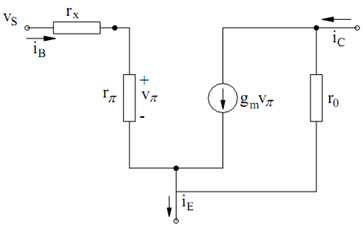## Hybrid Model of a Transistor Assignment Help

Assignment Help: >> Transistor Amplifier Circuits - Hybrid Model of a Transistor

Hybrid-π Model of a Transistor:

Let each voltage and current be written as the sum of a dc component and a small-signal ac component as follows :

iC  = IC  + ic                 iB  = I B  + ib

vBE  = VBE  + vbe          vCE  = VCE  + vce

If the ac components are adequately small, we can write

ic  =  (∂IC /∂VBE ) vbe +  ∂IC/∂Vce ;   ib =  ∂IB/∂VBE vbe

where the derivatives are evaluated at the dc bias values. Three useful small signal parameters of the BJT, known as its trans-conductance gm, the collector-to-emitter

resistance r0, and the base-to-emitter resistance rπ, can be defined now as follows :

gm        =  ∂IC    / ∂VBE = IS /VT  exp(VBE /VT    ) = IC /VT

ro  = (∂I/          ∂ VCE )-1  = [(ISO /VA  )exp (VBE /VT)]-1  = (VA+VCE/IC)

r π  = ( ∂I B  /∂VBE ) -1     = [ (ISO / β0 VT ) exp (VBE/ VT)]-1     = VT/IB

The collector and base currents can, thus, be written as

i c  = i′c + (v ce /r0  );      i  = vπ / rπ

where

ic′ = gm vπ ; vπ = vbe

The small-signal circuit which models these equations is specified in Figure. It is called the hybrid-π model. The resistor rx, which does not show in the above equations, is known as the base spreading resistance. It show the resistance of the connection to the base region inside the device. Since the base region is extremely narrow, the connection exhibits a resistance which frequently cannot be neglected.Figure: Hybrid π Model

The small-signal base-to-collector ac current gain β is described as the ratio  ic′ / ib. It is given by

β= ic′ /ib = gm vπ /ib  = gm   rπ = (IC  /VT )( VT / IB) = IC / IB

Note that ic differs from i′c by the current through r0. Therefore,   ic/ ib ≠ β , unless r0 = ∞.#### Assured A++ Grade

Get guaranteed satisfaction & time on delivery in every assignment order you paid with us! We ensure premium quality solution document along with free turntin report!

All rights reserved! Copyrights ©2019-2020 ExpertsMind IT Educational Pvt Ltd